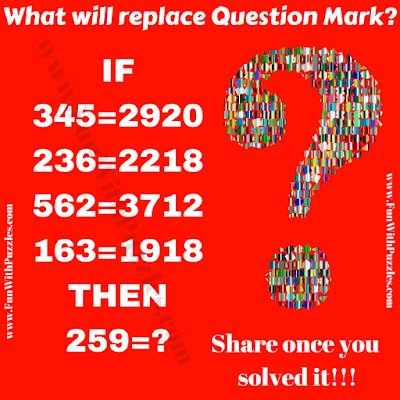Here is the Maths Question to blow your mind. In this Mind-Blowing Maths Question, there are some number equations. Your challenge is to find the logical reasoning for these equations which will make these equations correct. This Maths Question is targeted at school-going teens and adults. Kids will find this Puzzle very tough as it will require some complex Mathematical calculations. Let's see if you can solve this mind-blowing Maths Question and find the value of the missing number which will replace the question mark?Can you solve this Mind Blowing Maths Question?

The answer to this "Mind-Blowing Maths Question for Teens", can be viewed by clicking on the button. Please do give your best try before looking at the answer.

Unknown said...

240

Unknown said...

((4*5)+3^2)(4*5)=2920
((3*6)+2^2)(3*6)=2218
((6*2)+5^2)(6*2)=3712
((6*3)+1^2)(6*3)=1918
(5*9)+2^2)(5*9)=4945

Anonymous said...

4689

Rajesh Kumar said...

It will be great if you can write down your logical reasoning for solving this maths question.